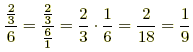index: click on a letter A B C D E F G H I J K L M N O P Q R S T U V W X Y Z A to Z index index: subject areas numbers & symbols sets, logic, proofs geometry algebra trigonometry advanced algebra & pre-calculus calculus advanced topics probability & statistics real world applications multimedia entrieswww.mathwords.com about mathwords website feedback

Fraction Rules

Algebra rules for combining fractions. These rules apply for both proper fractions and improper fractions. They apply for all rational expressions as well.

 A. Special Fractions 1.simplifies to b. 2.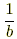does not simplify any further. 3.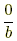simplifies to 0. 4.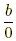is undefined. Examplesdoes not simplify.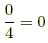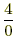is undefined. So is. Special note: Why is it OK to have 0 on top (in the numerator) and not on the bottom (in the denominator)? Consider for a moment what division means. The reason thatis because 2·5 = 10. The fractionbecause 2·0 = 0. The fraction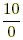can't equal anything. There is no number you can multiply by 0 and get 10 as your answer. The fractionis undefined. What about? It's undefined, too, but for a slightly different reason. If you multiply the 0 in the denominator by any number at all you get the 0 in the numerator. It seems thatcan equal any number. As a result we sayis indeterminate, which is a special kind of undefined expression. B. Negative Fractions 1.is the same asand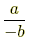2.simplifies to3.is NOT the same asExamples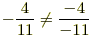C. Cancellation (a ≠ 0, b ≠ 0, c ≠ 0) 1.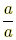cancels to 1 2.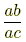cancels to3.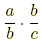cancels to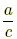4.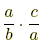cancels to5.cancels to b 6.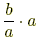cancels to b Examples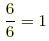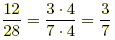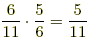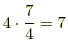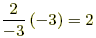D. Addition 1.2.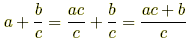3.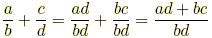Examples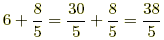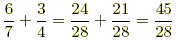E. Subtraction 1.2.3.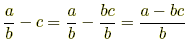4.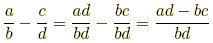Examples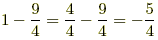F. Multiplication 1.2.3.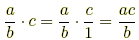Examples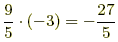Careful!! 1.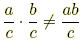2. Mixed numbers are shorthand for addition and not multiplication. For example,meansand NOT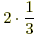. G. Division 1.2.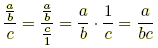3.Examples